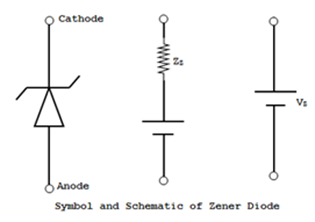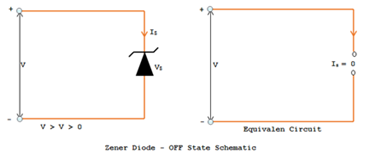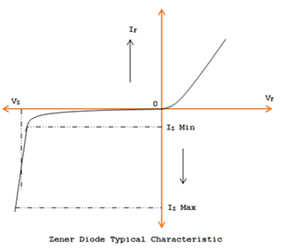# Zener Diode

Properly doped crystal diode which has a piercing breakdown voltage is called as Zener diode.

When the reverse bias on a PN junction crystal diode is enlarged, a critical voltage called as breakdown voltage is reached where the reverse current rises sharply to high value. The breakdown region is nothing but the knee of the reverse characteristic as shown in the figure.The suitable explanation of this breakdown junction was first given by the American scientist C. Zener. For that reason, the breakdown voltage is sometimes called as zener voltage and the sudden rise in current is termed as Zener current.

The breakdown voltage or Zener voltage depends upon the doping amount. If the diode is doped heavily, depletion layer will be thin and accordingly the breakdown of the junction will take place at lower reverse voltage.

On the other hand a lightly doped diode has a greater breakdown voltage. When a normal PN junction diode is appropriately doped so that it has a sharp breakdown voltage, it is called a zener diode.

### Symbol and schematic of Zener DiodeThe schematic symbol of a zener diode and its equivalent circuit are as shown in the figure (a). The complete equivalent circuit is shown in fig (b) and the approximate one in figure (c). Where it looks like a battery of Vz volts.

The schematics symbol of a zener diode is similar to that of a normal diode except that the line representing the cathode is bent at the both ends with a little mental effort, the cathode symbol can be imagined to look like the letter Z for zener.

### Zener Voltage

Zener diodes are available having zener voltage of 2.4V to 200V. This voltage is temperature dependent. Their power dissipation is given by the product of VzIz maximum ratings vary from 150mw to 50w.

#### The following points may be noted about zener diode:

• A zener diode is similar to an ordinary diode apart from that it is properly doped so as to make sure a sharp breakdown voltage.
• A zener diode at all times reverse connected i.e., it is always reverse biased.
• A zener diode has sharp breakdown voltage called zener voltage Vz.
• When it is in forward biased, their characteristics are just those of normal diode.
• The zener diode is not instantly burnt just because it has entered the breakdown region.

### Equivalent Circuit if Zener Diode

The analysis of circuits using zener diodes can be made quite easily by replacing zener diodes by its equivalent circuit.

#### ON State

When reverse voltage across a zener diode is equal to or more than breakdown voltage V, the current increases very sharply. In this region, the curve is almost vertical. It means that voltage across zener diode is constant at Vz even though the current through it changes.

Therefore in the breakdown region, an ideal can be represented by a battery of voltage Vz as shown in the fig. Under such conditions, the zener diode is said to be in the On state.

#### OFF State:When the reverse voltage across the zener diode is less than Vz but greater than 0 V, the zener diode is in the OFF state. Under such conditions, the zener diode can be represented by an open circuit as shown in the fig.

#### Uses of Zener Diode:

• As Voltage Regulator
• As a fixed reference voltage in a network for biasing and comparison purposes and for calibrating voltmeters.
• As peak clippers
• For meter protection against damage from accidental application of excessive voltage.

### VI Characteristics of Zener DiodeA typical characteristic is shown in the figure in the negative quadrant. The forward characteristic is simply that of an ordinary forward biased junction diode.

#### The important points on the reverse characteristics are

Vz = Zener Breakdown voltage
Izmin = Minimum current to sustain breakdown
Izmax = Maximum Zener current limited by maximum power dissipation
The reverse characteristic means that Vz remain constant eve when Iz increases considerably.1 CommentsComments

#### Read Comment Policy We have Zero Tolerance to Spam. Chessy Comments and Comments with Links will be deleted immediately upon our review.

1.It is okey, that Zener diode has ordinary characteristicin forward bias,
I'm just confused that how can a semiconductor diode can follows ohm's law ?

what is difference in ordinary and typical zener diode?